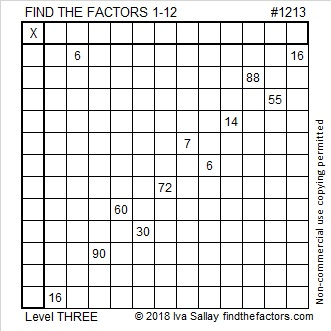# 1213 and Level 3

This puzzle would be a lot tougher to solve if I didn’t put the clues in the order that I did. Just start at the top of the puzzle and work your way down the puzzle clue by clue until you get to the bottom of the puzzle and have the entire thing solved.Print the puzzles or type the solution in this excel file: 12 factors 1211-1220

Now I’ll share a few facts about the number 1213:

• 1213 is a prime number.
• Prime factorization: 1213 is prime.
• The exponent of prime number 1213 is 1. Adding 1 to that exponent we get (1 + 1) = 2. Therefore 1213 has exactly 2 factors.
• Factors of 1213: 1, 1213
• Factor pairs: 1213 = 1 × 1213
• 1213 has no square factors that allow its square root to be simplified. √1213 ≈ 34.82815

How do we know that 1213 is a prime number? If 1213 were not a prime number, then it would be divisible by at least one prime number less than or equal to √1213 ≈ 34.8. Since 1213 cannot be divided evenly by 2, 3, 5, 7, 11, 13, 17, 19, 23, 29 or 31, we know that 1213 is a prime number.1213 is the sum of these nine consecutive prime numbers:
109 + 113 + 127 + 131 + 137 + 139 + 149 + 151 + 157 = 1213

27² + 22² = 1213
1213 is the hypotenuse of a Pythagorean triple:
245-1188-1213 calculated from 27² – 22², 2(27)(22), 27² + 22²

Here’s another way we know that 1213 is a prime number: Since its last two digits divided by 4 leave a remainder of 1, and 27² + 22² = 1213 with 27 and 22 having no common prime factors, 1213 will be prime unless it is divisible by a prime number Pythagorean triple hypotenuse less than or equal to √1213 ≈ 34.8. Since 1213 is not divisible by 5, 13, 17, or 29, we know that 1213 is a prime number.